﻿ 基于变分伴随的扩散方程系数反问题的数值反演算法 An Inversion Algorithm for Inverse Coefficient Problems in the Diffusion Equation Based on Variational Adjoint Method

Vol.07 No.04(2018), Article ID:24735,10 pages
10.12677/AAM.2018.74057

An Inversion Algorithm for Inverse Coefficient Problems in the Diffusion Equation Based on Variational Adjoint Method

Zhendong Wang, Gongsheng Li, Yingmei Wang

School of Mathematics and Statistics, Shandong University of Technology, Zibo Shandong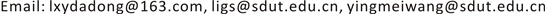Received: Apr. 12th, 2018; accepted: Apr. 23rd, 2018; published: Apr. 30th, 2018ABSTRACT

A numerical inversion algorithm is proposed based on the variational adjoint method for solving the backward problem and inverse source problem in the diffusion equation. With the help of the adjoint problem, a variational identity connecting the known data with the unknown is derived with which a linear system for the unknown is set forth by suitably choosing the solution of the adjoint equation. Numerical solutions to the inverse problems are obtained by solving the linear system with Tikhonov regularization, and numerical examples are presented to demonstrate the effectiveness of the inversion algorithm.

Keywords:Diffusion Equation, Inverse Problem, Adjoint Method, Variational Identity, Numerical Inversion1. 引言

2. 正问题与反问题

2.1. 正问题是带有(分片)光滑边界的有界开区域。对于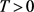，记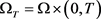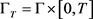，G为W的边界。考虑线性扩散方程：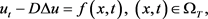(2.1)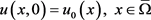(2.2)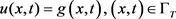(2.3)

2.2. 逆时问题与源项反问题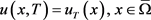(2.4)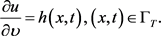(2.5)

3. 变分恒等式与反演算法

3.1. 变分恒等式(3.1)(3.2)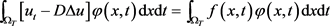(3.3)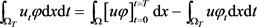(3.4)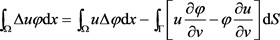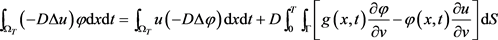(3.5)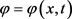是伴随问题(3.2)的解，则将式(3.4)与(3.5)代入(3.3)，并注意到初始函数与终值数据，整理即得(3.1)。证毕。

3.2. 反演算法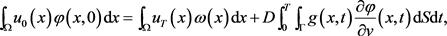(3.6)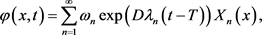(3.7)(3.8)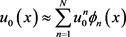(3.9)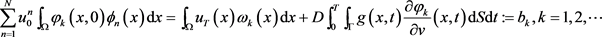(3.10)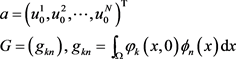(3.11)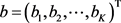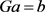(3.12)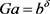(3.13)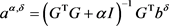(3.14)

4. 数值反演

4.1. 逆时问题的数值反演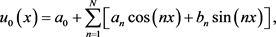(4.1)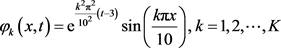(4.2)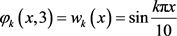。取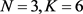，利用上一节所述的反演方法进行直接计算。图1绘制了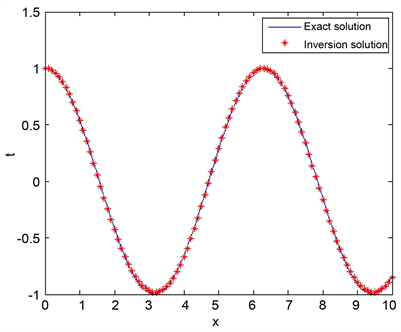Figure 1. Inversion result for the backward problem with accurate data in Ex.1.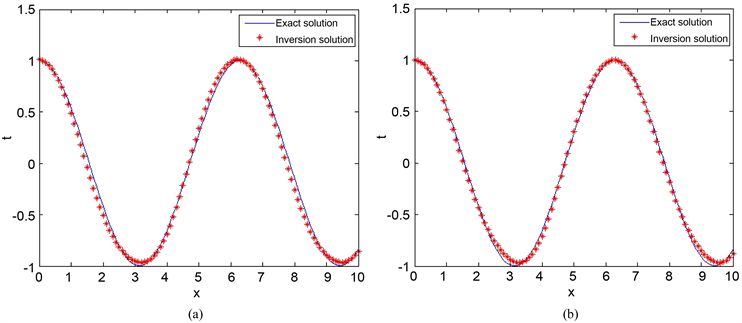Figure 2. Inversion result for the backward problem with noisy data in Ex.1. (a)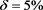; (b)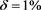4.2. 源项的数值反演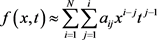(4.3)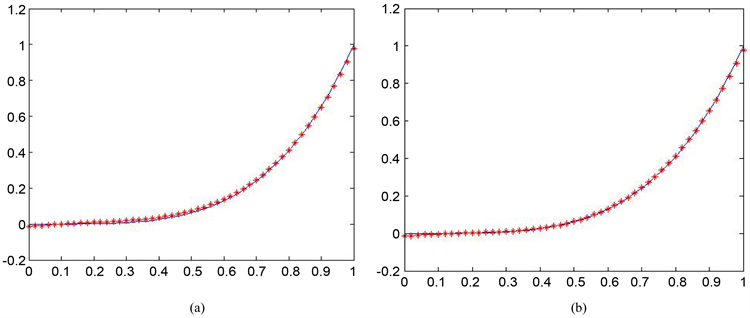Figure 3. Inversion results for the backward problem with noisy data in Ex.2. (a); (b)Figure 4. Exact source and inversion source solutions with accurate data in Ex.3. (a) Exact source; (b) Inversion source with accurate data(4.4)

5. 结论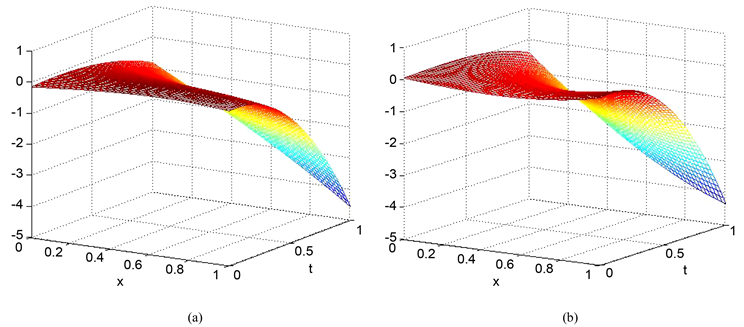Figure 5. The inversion source solutions with noisy data in Ex.3. (a); (b)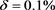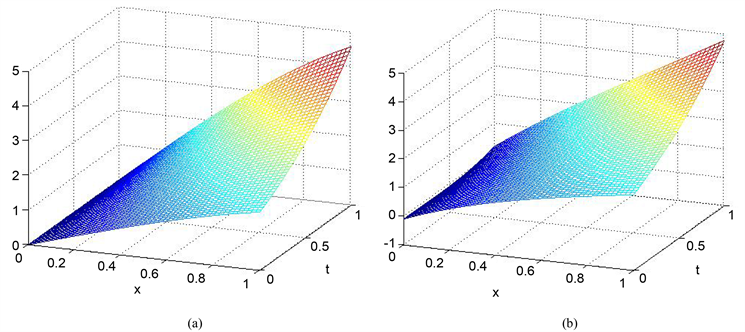Figure 6. Exact source and the inversion source solutions with accurate data in Ex.4. (a) Exact source; (b) Inversion source solution with accurate data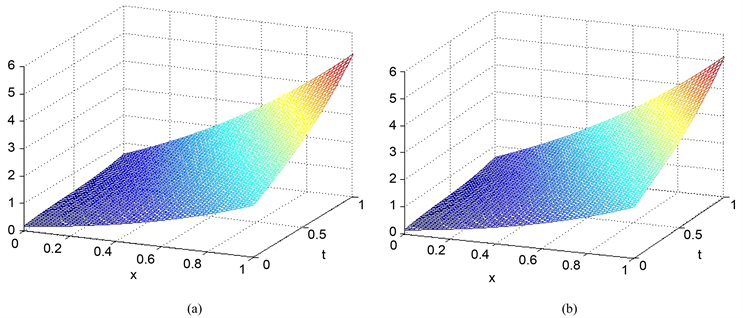Figure 7. Inversion source solutions with noisy data in Ex.4. (a); (b)An Inversion Algorithm for Inverse Coefficient Problems in the Diffusion Equation Based on Variational Adjoint Method[J]. 应用数学进展, 2018, 07(04): 466-475. https://doi.org/10.12677/AAM.2018.74057

1. 1. Cheng, J. and Yamamoto, M. (2000) The Global Uniqueness for Determining Two Convection Coefficients from Dirichlet to Neumann Map in Two Dimensions. Inverse Problems, 16, L25-L30. https://doi.org/10.1088/0266-5611/16/3/101

2. 2. DuChateau, P. (2013) An Adjoint Method for Proving Identifiability of Coefficients in Parabolic Equations. Journal of Inverse and Ill-Posed Problems, 21, 639-663. https://doi.org/10.1515/jip-2013-0010

3. 3. Isakov, V. (1998) Inverse Problems for Partial Differential Equations. Springer, New York. https://doi.org/10.1007/978-1-4899-0030-2

4. 4. Yamamoto, M. (2009) Carleman Estimates for Parabolic Equations and Applications. Inverse Problems, 25, 123013. https://doi.org/10.1088/0266-5611/25/12/123013

5. 5. Tikhonov, A.N., Goncharsky, A.V., Stepanov, V.V. and Yagola, A. (1995) Numerical Methods for the Solution of Ill-Posed Problems. Kluwer, Dordrecht. https://doi.org/10.1007/978-94-015-8480-7

6. 6. Engl, H.W., Hanke, M. and Neubauer, A. (1996) Regularization of Inverse Problems. Kluwer Academic, Dordrecht. https://doi.org/10.1007/978-94-009-1740-8

7. 7. 刘继军. 不适定问题的正则化方法及应用[M]. 北京: 科学出版社, 2005.

8. 8. 王彦飞. 反演问题的计算方法及其应用[M]. 北京: 高等教育出版社, 2007.

9. 9. 韩波, 李莉. 非线性不适定问题的求解方法及其应用[M]. 北京: 科学出版社, 2011.

10. 10. Cannon, J.R. (1984) The One Dimensional Heat Equation. Addison-Wesley, London.

11. 11. Beck, J.V., Blackwell, B. and St. Clair, C.R. (1985) Inverse Heat Conduction: Ill-Posed Problems. Wiley-Interscience, New York.

12. 12. Liu, C.-S. and Wang, P.F. (2016) An Analytic Adjoint Trefftz Method for Solving the Singular Parabolic Convection-Diffusion Equation and Initial Pollution Profile Problem. International Journal of Heat and Mass Transfer, 101, 1177-1184. https://doi.org/10.1016/j.ijheatmasstransfer.2016.05.134

13. 13. Liu, C.-S. (2016) A Simple Trefftz Method for Solving the Cauchy Problems of Three-Dimensional Helmholtz Equation. Engineering Analysis with Boundary Elements, 63, 105-113. https://doi.org/10.1016/j.enganabound.2015.11.009

14. 14. Liu, C.-S, Qu, W.Z. and Zhang, Y.M. (2018) Numerically Solving Twofold Ill-Posed Inverse Problems of Heat Equation by the Adjoint Trefftz Method. Numerical Heat Transfer: Part B, 73, 48-61. https://doi.org/10.1080/10407790.2017.1420317